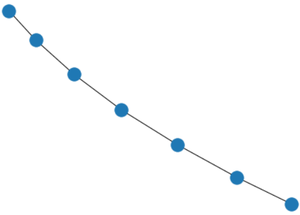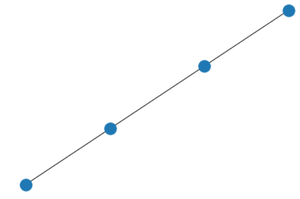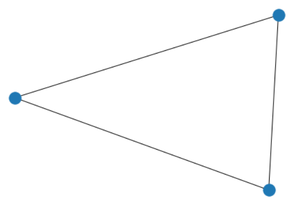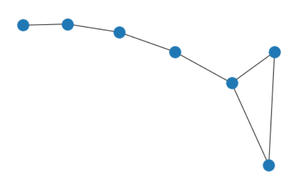Open in App
Not now

# Visualizing the composition two of graph using networkx

• Last Updated : 24 Jan, 2021

In this article, we are going to see how to visualize the composition two of the graph using networkx. If we are given two graphs A and B then their composition can be found by performing union operation on node-set and edge-set of A and B.

Simply if an edge is contained between any two vertices in any of the initial graphs it will be contained in the composition of those graphs.

Example:

Initial Graph G:G

Initial Graph H:H

Result of Composition of G and H:Composition(G,H)

Explanation: If we name nodes in both graphs G and H using integers then we will clearly observe that node and edge set of G will be superset of H and hence their union gives G in return.

Example 2:

Initial Graph G:G

Initial Graph H:H

Result of Composition of G and H:R

Explanation: We can see that all the edges which are in graph G are present in graph H except the node between first and third node from right therefore doing composition of G and H adds all edges from G and H into the result.

• H attributes take precedent over G attributes.
• The graph returned is of the same type as G.
• G and H should either be both directed or both undirected.
• G and H must both be graphs or multigraphs

Realizing Composition using Python :

We will use networkx.compose() function for our task to do composition of 2 graphs.

Syntax: compose(G, H)

• G: It is a networkx Graph object which is passed as an argument to compose function.
• H: It is also an networkx Graph object which is passed as an argument to compose function.

Result of compose function will be union of edge and vertices set of G and H.

Approach:

• We will import networkx with an alias nx.
• Create a sample graph object G using path_graph() function.
• Create second sample graph object H using path_graph() function.
• We will then call compose function passing G as an argument.
• We will capture the object returned by compose function in another object R.
• We will then call draw() function passing R as an argument which will display the complement graph.

Code:

## Python3

 `# importing networkx module ``import` `networkx as nx `` ` `# creating sample graph object ``G ``=` `nx.path_graph(``7``) `` ` `# creating sample graph object ``H ``=` `nx.path_graph(``3``)`` ` `# compose of G and H saving in R``R ``=` `nx.compose(G,H) `` ` `# calling draw() to visualize the complement graph ``nx.draw(R) `

Output:OUTPUT

My Personal Notes arrow_drop_up